# RD Sharma Solutions Chapter 4 Triangles Exercise 4.6 Class 10 Maths

 Chapter Name RD Sharma Chapter 4 Triangles Book Name RD Sharma Mathematics for Class 10 Other Exercises Exercise 4.1Exercise 4.2Exercise 4.3Exercise 4.4Exercise 4.5Exercise 4.7 Related Study NCERT Solutions for Class 10 Maths

### Exercise 4.5 Solutions

1. Triangles ABC and DEF are similar
(i) If area (ΔABC) = 16 cm2 , area(ΔDEF) = 25cm2 and  BC = 2.3 cm, find EF.
(ii) If area (ΔABC) = 9cm2 , area(ΔDEF) = 64 cm2 and DE = 5.1 cm, find AB.
(iii) If AC = 19 cm and DF = 8cm, find the ratio of the area of two triangles.
(iv) If area(ΔABC) = 36cm2 , area (ΔDEF) = 64 cm2 and DE = 6.2 cm, find AB.
(v) If AB = 1.2cm and DE = 1.4 cm, find the ratio of the areas of ΔABC and ΔDEF.

Solution

(i) If area (ΔABC) = 16 cm2 , area(ΔDEF) = 25cm2 and  BC = 2.3 cm, find EF.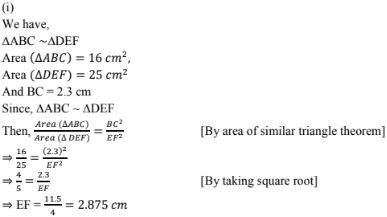(iii) If AC = 19 cm and DF = 8cm, find the ratio of the area of two triangles.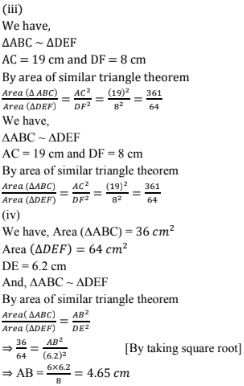2. In fig. below ΔACB ～ΔAPQ.  If BC = 10 m, PQ = 5cm, BA = 6.5 cm and AP = 2.8 cm, find CA and AQ. Also, find the area (ΔACB): area(ΔAPQ)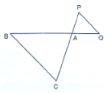Solution

3. The areas of two similar triangles are 81 cm2 and 49cm2  respectively. Find the ratio of their corresponding heights. What is the ratio of their corresponding medians ?

Solution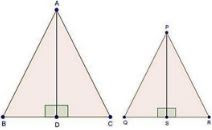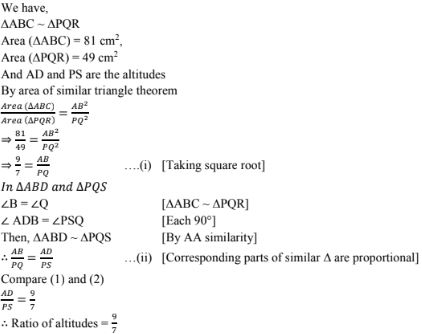Since, the ratio of the area of two similar triangles is equal to the ratio of the squares of the squares of their corresponding altitudes and is also equal to the squares of their corresponding medians.
Hence, ratio of altitudes = Ratio of medians = 9 : 7

4. The areas of two similar triangles are 169 cm2 and 121 cm2 respectively. If the longest side of the larger triangle is 26 cm, find the longest side of the smaller triangle.

Solution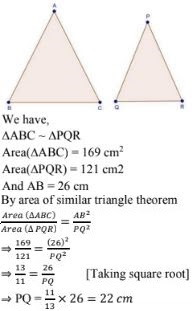5. Two isosceles triangles have equal vertical angles and their areas are in the ratio 36 : 25. Find the ratio of their corresponding heights.
Solution

6. The areas of two similar triangles are 25 cm2 and 36cm2 respectively. If the altitude of the first triangle 2.4 cm, find the corresponding altitude of the other.
Solution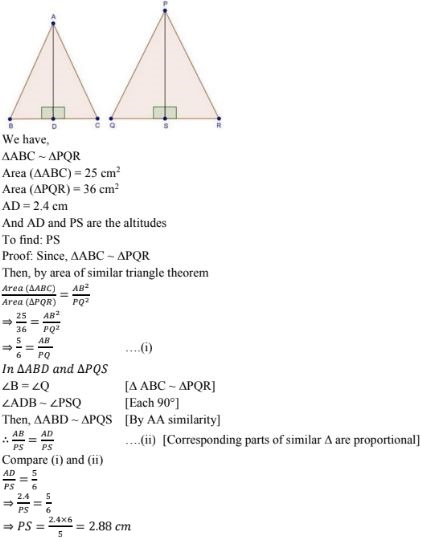7. The corresponding altitudes of two similar triangles are 6 cm an 9cm respectively. Find the ratio of their areas.
Solution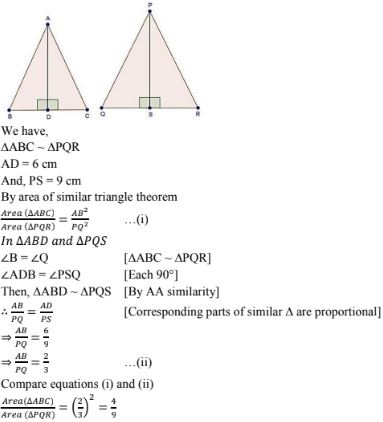8. ABC is a triangle n which ∠A = 90° , AN ⊥ BC,  BC = 12 cm and AC = 5 cm . Find the ratio of the areas of ΔANC and ΔABC.
Solution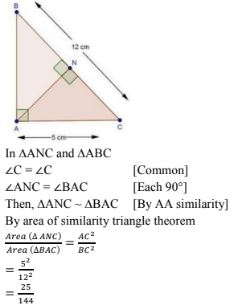9. In Fig. 4.178, DE ||BC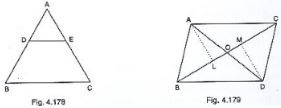(i) If DE = 4cm, BC = 6cm and Area (ΔADE) = 16 cm2 , find the area of ΔABC.
(ii) If DE = 4cm, BC = 8cm and Area (ΔADE) = 25 cm2 , find the area of ΔABC.
(iii) If DE : BC = 3 : 5. Calculate the ratio of the areas of ΔADE and the trapezium BCED.
Solution

We have, DE||BC, DE = 4cm, BC = 6 cm and area(ΔADE) = 16cm2
∠A = ∠A  [Common]
Then, ΔADE ～ΔABC  [By AA similarity]
∴ By area of similar triangle theorem

10. In ΔABC, D and E are the mid - Points of AB and AC respectively. Find the ratio of the areas of ΔADE and ΔABC
Solution
11. In Fig., 4.179, ΔABC and ΔDBC are on the same base BC. If AD and BC intersect at O, prove that area(ΔABC)/area(ΔDBC) = AO/DO
Solution
We know that area of a triangle = 1/2 × Base × height
Since ΔABC and ΔDBC are one same base.
Therefore ratio between their areas will be as ratio of their heights.
Let us draw two perpendiculars AP and DM on line BC>

12. ABCD is a trapezium in which AB||CD. The diagonals AC and BD intersect at O. Prove that :
(i) ΔAOB and ΔCOD
(ii) If OA = 6 cm, OC = 8cm,
Find :
(a) area(ΔAOB)/area(ΔCOD)
(b) area(ΔAOD)/area(ΔCOD)
Solution

13. In ABC, P divides the side AB such that AP : PB = 1 : 2. Q is a point in AC such that PQ || BC. Find the ratio of the areas of ΔAPQ and trapezium BPQC.
Solution

14. The areas of two similar triangles are 100 cm2 and 49 cm2 respectively. If the altitude the bigger triangle is 5 cm, find the corresponding altitude of the other.
Solution

15. The areas of two similar triangles are 121cm2 and 64 cm2 respectively. If the median of the first triangle is 12.1 cm, find the corresponding median of the other.
Solution

16. If ΔABC ～ΔDEF such that AB = 5cm, area(ΔABC) = 20 cm2 and area (ΔDEF) = 45cm2 , determine DE.
Solution
We have,
ΔABC ～ΔDEF such that AB = 5cm,
Area(ΔABC) = 20cm2 and area(ΔDEF) = 45cm2
By area of similar triangle theorem

17. In ΔABC, PQ is a line segment intersecting AB at P and AC at Q such hat PQ || BC and PQ divides ΔABC into two parts equal in area. Find BP/AB.
Solution

18. The areas of two similar triangles ABC and PQR are in the ratio 9 : 16. If BC = 4.5 cm, find the length of QR.
Solution
We have

19. ABC is a triangle and PQ is a straight line meeting AB in P and AC in Q. if AP = 1 cm, PB = 3 cm, AQ = 1.5cm, QC = 4.5m, prove that area of ΔAPQ is one - sexteenth of the area of ABC.
Solution

20. If D is a point on the side AB of ΔABC such that AD : DB = 3 : 2 and E is a Point of BC such that DE || AC. Find the ratio of areas of ΔABC and ΔBDE.
Solution

21. If ∆ABC and ∆BDE are equilateral triangles, where D is the mid-point of BC, find the ratio of areas of ∆ABC and ∆BDE.
Solution

22. AD is an altitude of an equilateral triangle ABC. On AD as base, another equilateral triangle ADE is constructed. Prove that area (∆ADE) : area(∆ABC) = 3 : 4
Solution